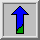# Spatial Domain

For simplicity, assume that the image I being considered is formed by projection from scene S (which might be a two- or three-dimensional scene, etc.).

The spatial domain is the normal image space, in which a change in position in I directly projects to a change in position in S. Distances in I (in pixels) correspond to real distances (e.g. in meters) in S.

This concept is used most often when discussing the frequency with which image values change, that is, over how many pixels does a cycle of periodically repeating intensity variations occur. One would refer to the number of pixels over which a pattern repeats (its periodicity) in the spatial domain.

In most cases, the Fourier Transform will be used to convert images from the spatial domain into the frequency domain.

A related term used in this context is spatial frequency, which refers to the (inverse of the) periodicity with which the image intensity values change. Image features with high spatial frequency (such as edges) are those that change greatly in intensity over short image distances.

Another term used in this context is spatial derivative, which refers to how much the image intensity values change per change in image position.